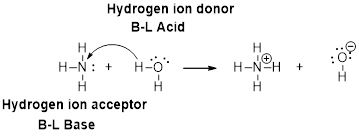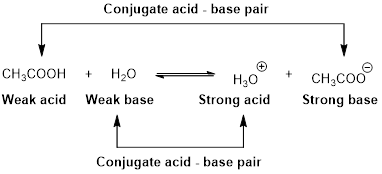# In each of the following acid-base reactions, identify the Brønsted acid and base on the left and their conjugate partners on the right. (a) HCO 2 H(aq) + H 2 O( ℓ ) ⇄ HCO 2 − (aq) + H 3 O + (aq) (b) NH 3 (aq) + H 2 S(aq) ⇄ NH 4 + (aq) + HS − (aq) (c) HSO 4 − (aq) + OH − (aq) ⇄ SO 4 2− (aq) + H 2 O + ( ℓ )### Chemistry & Chemical Reactivity

9th Edition
John C. Kotz + 3 others
Publisher: Cengage Learning
ISBN: 9781133949640

#### Solutions

Chapter
Section### Chemistry & Chemical Reactivity

9th Edition
John C. Kotz + 3 others
Publisher: Cengage Learning
ISBN: 9781133949640
Chapter 16, Problem 7PS
Textbook Problem
46 views

## In each of the following acid-base reactions, identify the Brønsted acid and base on the left and their conjugate partners on the right. (a) HCO2H(aq) + H2O(ℓ) ⇄ HCO2−(aq) + H3O+(aq) (b) NH3(aq) + H2S(aq) ⇄ NH4+(aq) + HS−(aq) (c) HSO4−(aq) + OH−(aq) ⇄ SO42−(aq) + H2O+(ℓ)

(a)

Interpretation Introduction

Interpretation:

Bronsted acid and base on the left and their conjugate partners on the right has to be identified.

HCO2H (aq) + H2O (l) HCO2- (aq) + H3O+(aq)

Concept Introduction:

Bronsted –Lowry definitions:

A Bronsted –Lowry acid is a proton donor, it donates a hydrogen ion, H+

A Bronsted-Lowry base is a proton acceptor, it accepts a hydrogen ion H+.Bronsted –Lowry conjugate acid-Base pairs:

When an acid is dissolved in water, the acid (HA) donates a proton to water to form a new acid (conjugate acid) and a new base (conjugate base).### Explanation of Solution

HCO2H (aq) + H2O (l) HCO2- (aq) + H3O+(aq)

In the above reaction,

HCO2H is donated a proton to H2O. Therefore, HCO2H is a Bronsted acid.

H2O is accepted a proton from HCO2H. Therefore, H2O is a Bronsted base.

The conjugate base of HCO2H is HCO2 because HCO2 has one proton less than HCO2H

(b)

Interpretation Introduction

Interpretation:

Bronsted acid and base on the left and their conjugate partners on the right has to be identified.

NH3(aq) + H2S (aq) NH4+ (aq) + HS-(aq)

Concept Introduction:

Bronsted –Lowry definitions:

A Bronsted –Lowry acid is a proton donor, it donates a hydrogen ion, H+

A Bronsted-Lowry base is a proton acceptor, it accepts a hydrogen ion H+.Bronsted –Lowry conjugate acid-Base pairs:

When an acid is dissolved in water, the acid (HA) donates a proton to water to form a new acid (conjugate acid) and a new base (conjugate base).(c)

Interpretation Introduction

Interpretation:

Bronsted acid and base on the left and their conjugate partners on the right has to be identified.

HSO4-(aq) + OH- (aq) SO42-(aq) + H2O (l)

Concept Introduction:

Bronsted –Lowry definitions:

A Bronsted –Lowry acid is a proton donor, it donates a hydrogen ion, H+

A Bronsted-Lowry base is a proton acceptor, it accepts a hydrogen ion H+.Bronsted –Lowry conjugate acid-Base pairs:

When an acid is dissolved in water, the acid (HA) donates a proton to water to form a new acid (conjugate acid) and a new base (conjugate base).### Still sussing out bartleby?

Check out a sample textbook solution.

See a sample solution

#### The Solution to Your Study Problems

Bartleby provides explanations to thousands of textbook problems written by our experts, many with advanced degrees!

Get Started

Find more solutions based on key concepts
The best and safest source of vitamin D for people in the United States is exposure to sunshine. T F

Nutrition: Concepts and Controversies - Standalone book (MindTap Course List)

What is the difference between the Milky Way and the Milky Way Galaxy?

Horizons: Exploring the Universe (MindTap Course List)

Review. Why is the following situation impossible? An athlete tests her hand strength by having an assistant ha...

Physics for Scientists and Engineers, Technology Update (No access codes included)

How are organisms named?

Oceanography: An Invitation To Marine Science, Loose-leaf Versin